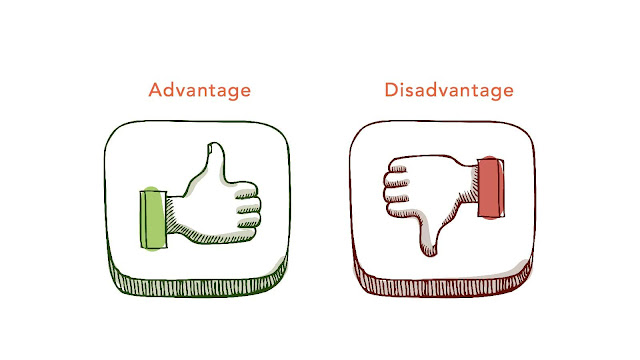# HSSlive: Plus One & Plus Two Notes & Solutions for Kerala State Board

## Monday, March 13, 2023Advantages And Disadvantages Of 2D Rectangular Matrix

## What is 2D Rectangular Matrix?

A 2D rectangular matrix, also known as a two-dimensional rectangular array or simply a matrix, is a collection of elements arranged in rows and columns. It is called "rectangular" because all the rows have the same number of elements, and all the columns have the same number of elements.

For example, the following is a 2D rectangular matrix:

`1 2 3`
`4 5 6 `
`7 8 9 `

This matrix has three rows and three columns, and each element is identified by its row and column indices. The element in the first row and second column is 2, and the element in the third row and third column is 9.

2D rectangular matrices are commonly used in computer science and mathematics for a variety of purposes, such as representing images, storing data, and performing matrix operations.

## Advantages of 2D Rectangular Matrix

There are several advantages to using a 2D rectangular matrix:

1. Easy to understand: The 2D rectangular matrix is easy to understand because it visually represents data in a grid-like format. This makes it easy to identify patterns and relationships between the data.

2. Efficient access to elements: Accessing an element in a 2D rectangular matrix is efficient because it requires only two indices, one for the row and one for the column. This makes it easy to perform operations on specific elements or subsets of elements.

3. Useful for mathematical operations: 2D rectangular matrices are useful for mathematical operations such as matrix multiplication, matrix addition, and matrix inversion. These operations are commonly used in fields such as computer graphics, machine learning, and engineering.

4. Compact representation of data: A 2D rectangular matrix can represent a large amount of data in a compact format. This is useful for storing and manipulating data in computer programs.

5. Supports spatial operations: 2D rectangular matrices are commonly used to represent spatial data such as images and maps. This is because the matrix format supports operations such as rotation, scaling, and translation, which are important for spatial analysis and visualization.

## Disadvantages of 2D Rectangular Matrix

While 2D rectangular matrices have several advantages, there are also some disadvantages to consider:

1. Fixed size: 2D rectangular matrices have a fixed size, which means they cannot be resized dynamically. This can be problematic if the size of the data changes frequently or if the size is unknown in advance.

2. Inefficient for sparse data: If a significant portion of the data is missing, a 2D rectangular matrix can be inefficient because it requires space to be allocated for all the elements, even those that are empty.

3. Not suitable for irregular data: If the data is irregular, a 2D rectangular matrix may not be suitable for representing it. For example, if the data has varying lengths for each row or column, a rectangular matrix may not be able to represent it efficiently.

4. Complexity: Performing complex operations on 2D rectangular matrices can be computationally expensive and time-consuming, especially for large matrices.

5. Overhead: A 2D rectangular matrix requires additional memory overhead to store the row and column indices of each element, which can be a concern for large matrices.

Overall, while 2D rectangular matrices have many benefits, they are not always the most efficient or appropriate data structure for every use case.

Share: